Anzeige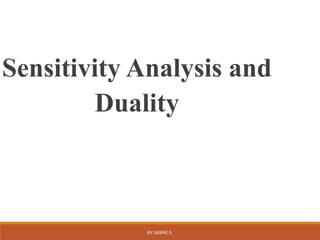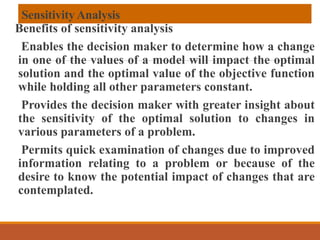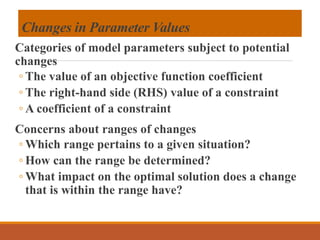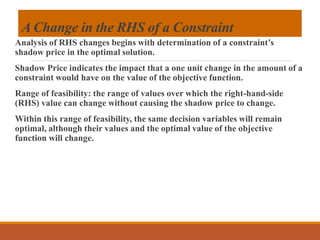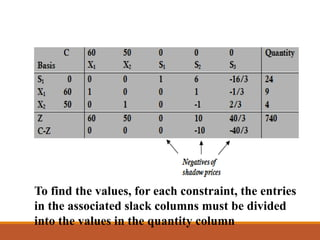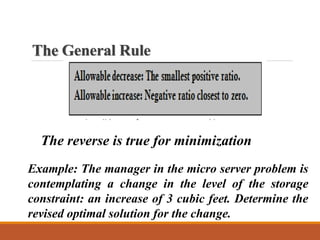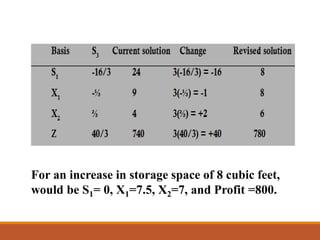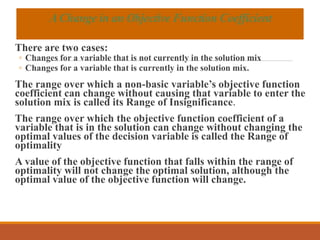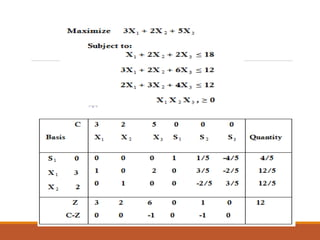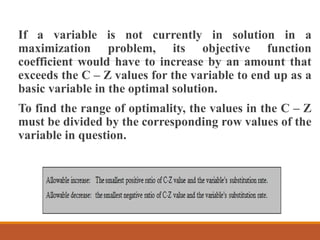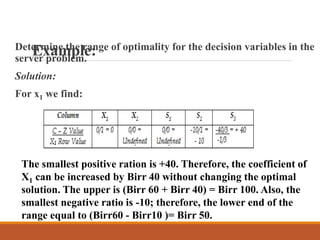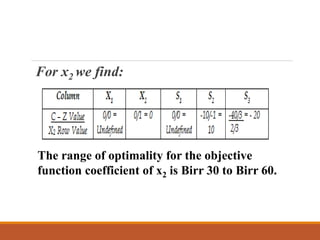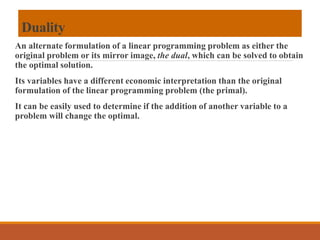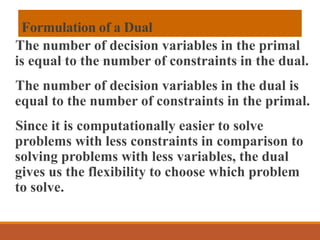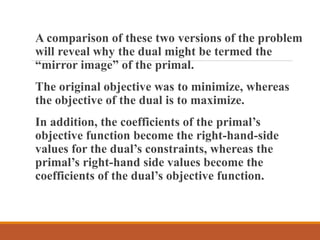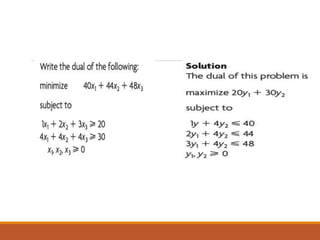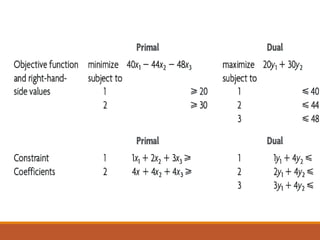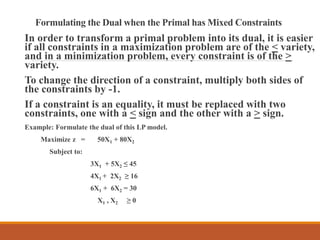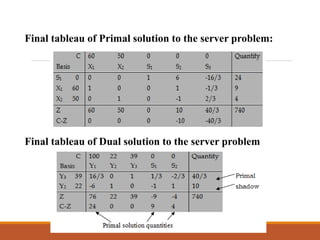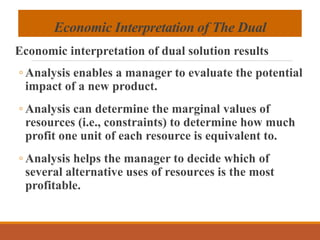1 von 21
Anzeige

### chapter 2 post optimality.pptx

1. BY GEBRE S. Sensitivity Analysis and Duality
2. SensitivityAnalysis Benefits of sensitivity analysis Enables the decision maker to determine how a change in one of the values of a model will impact the optimal solution and the optimal value of the objective function while holding all other parameters constant. Provides the decision maker with greater insight about the sensitivity of the optimal solution to changes in various parameters of a problem. Permits quick examination of changes due to improved information relating to a problem or because of the desire to know the potential impact of changes that are contemplated.
3. Changes in Parameter Values Categories of model parameters subject to potential changes ◦ The value of an objective function coefficient ◦ The right-hand side (RHS) value of a constraint ◦ A coefficient of a constraint Concerns about ranges of changes ◦ Which range pertains to a given situation? ◦ How can the range be determined? ◦ What impact on the optimal solution does a change that is within the range have?
4. A Change in the RHS of a Constraint Analysis of RHS changes begins with determination of a constraint’s shadow price in the optimal solution. Shadow Price indicates the impact that a one unit change in the amount of a constraint would have on the value of the objective function. Range of feasibility: the range of values over which the right-hand-side (RHS) value can change without causing the shadow price to change. Within this range of feasibility, the same decision variables will remain optimal, although their values and the optimal value of the objective function will change.
5. The final simplex table for server problem To find the values, for each constraint, the entries in the associated slack columns must be divided into the values in the quantity column
6. The General Rule The reverse is true for minimization Example: The manager in the micro server problem is contemplating a change in the level of the storage constraint: an increase of 3 cubic feet. Determine the revised optimal solution for the change.
7. Solution For an increase in storage space of 8 cubic feet, would be S1= 0, X1=7.5, X2=7, and Profit =800.
8. There are two cases: ◦ Changes for a variable that is not currently in the solution mix ◦ Changes for a variable that is currently in the solution mix. The range over which a non-basic variable’s objective function coefficient can change without causing that variable to enter the solution mix is called its Range of Insignificance. The range over which the objective function coefficient of a variable that is in the solution can change without changing the optimal values of the decision variable is called the Range of optimality A value of the objective function that falls within the range of optimality will not change the optimal solution, although the optimal value of the objective function will change. AChange in an Objective Function Coefficient
9. If a variable is not currently in solution in a maximization problem, its objective function coefficient would have to increase by an amount that exceeds the C – Z values for the variable to end up as a basic variable in the optimal solution. To find the range of optimality, the values in the C – Z must be divided by the corresponding row values of the variable in question.
10. Example: Determine the range of optimality for the decision variables in the server problem. Solution: For x1 we find: The smallest positive ration is +40. Therefore, the coefficient of X1 can be increased by Birr 40 without changing the optimal solution. The upper is (Birr 60 + Birr 40) = Birr 100. Also, the smallest negative ratio is -10; therefore, the lower end of the range equal to (Birr60 - Birr10 )= Birr 50.
11. For x2 we find: The range of optimality for the objective function coefficient of x2 is Birr 30 to Birr 60.
12. Duality An alternate formulation of a linear programming problem as either the original problem or its mirror image, the dual, which can be solved to obtain the optimal solution. Its variables have a different economic interpretation than the original formulation of the linear programming problem (the primal). It can be easily used to determine if the addition of another variable to a problem will change the optimal.
13. Formulation of a Dual The number of decision variables in the primal is equal to the number of constraints in the dual. The number of decision variables in the dual is equal to the number of constraints in the primal. Since it is computationally easier to solve problems with less constraints in comparison to solving problems with less variables, the dual gives us the flexibility to choose which problem to solve.
14. A comparison of these two versions of the problem will reveal why the dual might be termed the “mirror image” of the primal. The original objective was to minimize, whereas the objective of the dual is to maximize. In addition, the coefficients of the primal’s objective function become the right-hand-side values for the dual’s constraints, whereas the primal’s right-hand side values become the coefficients of the dual’s objective function.
15. Example
16. Table: Transforming the Primal into Its Dual
17. Exercise Formulate the dual of the server problem
18. Formulating the Dual when the Primal has Mixed Constraints In order to transform a primal problem into its dual, it is easier if all constraints in a maximization problem are of the < variety, and in a minimization problem, every constraint is of the > variety. To change the direction of a constraint, multiply both sides of the constraints by -1. If a constraint is an equality, it must be replaced with two constraints, one with a < sign and the other with a > sign. Example: Formulate the dual of this LP model. Maximize z = 50X1 + 80X2 Subject to: 3X1 + 5X2 ≤ 45 4X1 + 2X2 ≥ 16 6X1 + 6X2 = 30 X1 , X2 ≥ 0
19. Final tableau of Primal solution to the server problem: Final tableau of Dual solution to the server problem
20. Economic Interpretation of The Dual Economic interpretation of dual solution results ◦ Analysis enables a manager to evaluate the potential impact of a new product. ◦ Analysis can determine the marginal values of resources (i.e., constraints) to determine how much profit one unit of each resource is equivalent to. ◦ Analysis helps the manager to decide which of several alternative uses of resources is the most profitable.
Anzeige